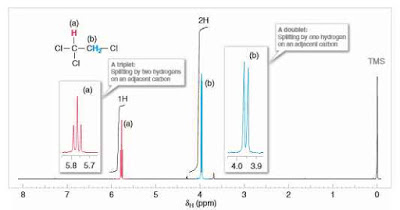# Integration of Signal Areas:

The area under each signal in a 1H NMR spectrum is proportional to the number of hydrogen atoms producing that signal.

In the 1H NMR spectrum of 1,4-dimethylbenzene (Fig. 9.3), you may have noticed curves that resemble steps over each signal. The height of each step (using any unit of measure) is proportional to the area of the NMR signal underneath it, and also to the number of hydrogen atoms giving rise to that signal.

The area under each signal (shown with blue shading above) is what is measured (integrated) and taken as a ratio to compare the relative numbers of hydrogen atoms producing each signal in an NMR spectrum. We have indicated the relative step heights as 1.0 and 1.5 (in dimensionless units). Had these values not been given, we would have measured the step heights with a ruler and taken their ratio.

Since the actual number of hydrogen atoms giving rise to the signals is not likely to be 1 and 1.5 (we cannot have a fraction of an atom), we can surmise that the true number of hydrogens producing the signals is probably 2 and 3, or 4 and 6, etc. For 1,4-dimethylbenzene the actual values are, of course, 4 and 6. Whether NMR data are provided  with an integral step over each signal, or simply with numbers that represent each signal’s relative area, the process of interpreting the data is the same because the area of each signal is proportional to the number of hydrogen atoms producing that signal. (It is important to note that in 13CNMR spectroscopy signal area is not relevant in routine analyses.)

## Coupling (Signal Splitting):

Coupling, also referred to as signal splitting or signal multiplicity, is a third feature of 1H NMR spectra that provides very useful information about the structure of a compound.

Coupling is caused by the magnetic effect of nonequivalent hydrogen atoms that are within 2 or 3 bonds of the hydrogens producing the signal The effect of the nearby hydrogens is to split (or couple with) the energy levels of the hydrogens whose signal is being observed, and the result is a signal with multiple peaks. (Notice that we have been careful to differentiate use of the words signal and peak. A group of equivalent atoms produces one signal that may be split into multiple peaks.)

We shall explain the physical origin of coupling further in Section 9.9; however, the importance of coupling is that it is predictable, and it gives us speciﬁc information about the constitution of the molecule under study.

The typical coupling we observe is from nonequivalent, vicinal hydrogens, that is, from hydrogens on adjacent carbons, separated by three bonds from the hydrogens producing the signal. Coupling can also occur between nonequivalent geminal hydrogens (hydrogens bonded to the same carbon) if the geminal hydrogens are in a chiral or conformationally restricted molecule.

A simple rule exists for predicting the number of peaks expected from vicinal coupling in 1H NMR:

This rule is applicable in general to achiral molecules without conformational barriers. The 1H NMR spectrum of 1,4-dimethylbenzene  is an example where n  0 (in the above equation) regarding the hydrogen atoms producing the signals at d 7.0 and at d 2.3. There are no hydrogen atoms on the carbons adjacent to the methyl groups; hence n  0 for the signal at d 2.3, and the signal is a singlet (signals with only one peak are called singlets).

And, since all of the hydrogen atoms on the ring are equivalent by symmetry and there are no adjacent nonequivalent hydrogen atoms, n  0 for the hydrogens on the ring producing the signal at d 7.0, and hence this signal is a singlet as well.

The 1H NMR spectrum of 1,1,2-trichloroethane, shown in Fig. 9.4, provides an example where n is not equal to zero, and coupling is therefore evident. In the spectrum of 1,1,2-trichloroethane we see two signals: one with three peaks and one with two peaks.

These signals are called, respectively, a triplet and a doublet. The signal for the CHCl2 hydrogen is a triplet because there are two hydrogen atoms on the adjacent carbon (n =2). The signal for the CH2Cl hydrogens is a doublet because there is one hydrogen on the adjacent carbon (n=1).Integration of Signal Areas in HNMR-Coupling or Signal Splitting